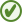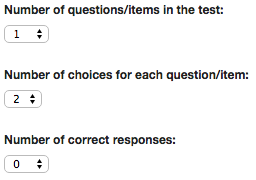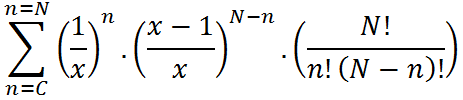### Categorisation

Choose the classification system you would like to use:

# Work out how likely an assessment result could have been achieved by chanceEarly years skill: Any Early years typical range: Any P-scales/Curriculum skill: Any P-scales/Curriculum level: Any TAP skill: Any TAP level: Any Pre/Nat. Curriculum Area: not specified Pre/Nat. Curiculum Standard: not specified Phonology/Articulation: Any
 Section: Early Years (0-5yrs) info; Primary (5-11yrs) info; Secondary (11-16yrs) info; Post School Education info; Adult info
If you are having problems with logging into the site, please email us on support@commtap.org or give us a call.
A calculator that you can use to work out how likely that a child/adult could have come up with a score in an assessment test by chance. For example, suppose you had 12 pairs of pictures, and a child was able to point to 9 of them correctly from an instruction - is that a good result or not? No hard maths involved!Multiple Choice (Assessment) Probability Calculator

As speech and language therapists and other professionals, we carry out assessments to find out what language clients are able to understand. This will help us to determine what types of assistance they may need, and what types of communication strategies might be useful (which could include using alternative forms of communication such as communication aids, signing).

Where we have only been able to test a particular skill using a small number of items, the probability that a client could have done well just by chance will be greater - and could lead to an over-optimistic evaluation of their ability. This could particularly happen where people with significant physical difficulties are being assessed - for example due to physical constraints they may only be able to indicate a choice between two pictures, and they may tire easily.

Suppose, for example, you design a test to look at a client's understanding of the names of day to day objects which has twelve items (presentations) in it, and for each item the client chooses between two pictures. Suppose they get 9 out of the 12 items correct. How likely is it then that they actually do have an understanding of this type of language, and how likely is it that they could have just got this number correct if they were choosing at random? How about if they got 8 out of 12? Or 10 out of 12?

It turns out that the probability of getting 9 or more out of 12 in this test purely by chance is 7% - meaning that if you carried out this test lots of times and tossed a coin to choose the responses, 7% of the times you did the test, you would get a score of 9 or greater. For 10 or more out of 12, chance is 2%, and for 8 or more out of 12, it would be 19%.

You can work this probability out using Commtap's Multiple Choice Probability Calculator.

## The maths

The formula we use to work this out is:Where:

N is the total number of items in the test;

x is the number of choices for each item;

C is the number of correct responses.

### What the maths means

Let's imagine we did a test which had seven items, for each item the person could choose from four pictures, and they got three out of the seven right.

With four pictures, the probability of getting one item right by chance is 1/x or 1/4 in our example and the probability of getting it wrong by chance is ((x-1)/x) or 3/4 here. They got three items right, and four items wrong. The probability of them getting exactly the three items they chose right and the other four items wrong is (1/4) multiplied by itself three times multiplied by (3/4) four times - 1/64 x 81/256 which is 81/16384 or 0.00494384765625.

There are other ways of getting this number of items right - the bit at the end of the formula is the number of ways of getting this number of items right (N!/(n!(N-n)!) - in this case it's (7 x 6 x 5 x 4 x 3 x 2 x 1)/((3 x 2 x 1) x (4 x 3 x 2 x 1)) = 35 ways of getting 3 out of 7 items right. (More about maths combinations). You could count them to check this is the case! This gives the probability of getting exactly three items right by chance as 0.173 (or 17.3%).

To get the probability that three or more items could have been correct by chance we need to add this probability to the chance of getting four items right, five items right, six items right and seven items right. The "E" at the beginning of the formula means work out the probability for all of these and add them together. If we do that, we get a probability for this example of 0.243591 or 24.3591% (that's about a 1 in 4 chance): you're about 75% sure that the result of this test is not a chance result (so not that sure in this case).If you find the Commtap site useful, please fill out a review of it on EdTech impact. This really helps us to get funding to continue running the site. Thank you!